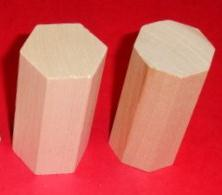## Wednesday, October 26, 2011

### Geometry - What is a Prism?

A PRISM is a 3D Shape or Geometric Solid.
Features: A Prism has 3 or more rectangular faces and 2   identical parallel bases.Rectangular PrismRectangular Prism
Examples:
• Rectangular Prisms - made of 6 rectangular faces.
• Triangular Prisms - made of 3 rectangular faces and 2 triangular bases
• Pentagonal Prisms - made of 5 rectangular faces and 2 pentagonal bases
• Hexagonal Prism - made of 6 rectangular faces and 2 hexagonal bases
• Octagonal Prism - made of 8 rectangular faces and 2 octagonal bases
• Etc.

Suggested Activities

• Have students observe prism manipulatives or pictures of a prisms.  Discuss their properties. Have students describe the features of different prisms.Triangular PrismHexagonal Prism and Octagonal Prism

• Have students look in their homes or classrooms for examples of prisms. How many can they find?Rectangular Prism

• Have students create prisms with Arts and Crafts Materials.
• Have students create prisms with NETS  (2D representations of 3D figures).Rectangular PrismTriangular Prism

Have fun with math!

____________________________________________________